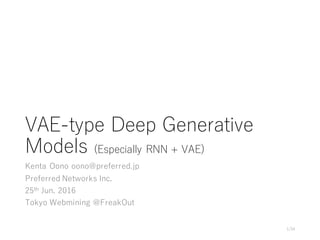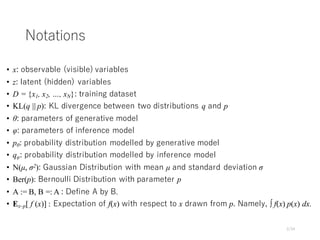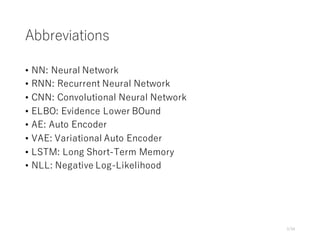Successfully reported this slideshow.

# VAE-type Deep Generative Models×

1 of 34
1 of 34

# VAE-type Deep Generative Models

Talk in Tokyo Webmining @FreakOut

Talk in Tokyo Webmining @FreakOut

### VAE-type Deep Generative Models

1. 1. VAE-type Deep Generative Models (Especially RNN + VAE) Kenta Oono oono@preferred.jp Preferred Networks Inc. 25th Jun. 2016 Tokyo Webmining @FreakOut 1/34
2. 2. Notations • x: observable (visible) variables • z: latent (hidden) variables • D = {x1, x2, …, xN}: training dataset • KL(q || p): KL divergence between two distributions q and p • θ: parameters of generative model • φ: parameters of inference model • pθ: probability distribution modelled by generative model • qφ: probability distribution modelled by inference model • N(µ, σ2): Gaussian Distribution with mean µ and standard deviation σ • Ber(p): Bernoulli Distribution with parameter p • A := B, B =: A : Define A by B. • Ex~p[ f (x)] : Expectation of f(x) with respect to x drawn from p. Namely, ∫ f(x) p(x) dx. 2/34
3. 3. Abbreviations • NN: Neural Network • RNN: Recurrent Neural Network • CNN: Convolutional Neural Network • ELBO: Evidence Lower BOund • AE: Auto Encoder • VAE: Variational Auto Encoder • LSTM: Long Short-Term Memory • NLL: Negative Log-Likelihood 3/34
4. 4. Agenda • Mathematical formulation of generative models. • Variational Auto Encoder (VAE) • Variants of VAE • RVAE, VRNN, DRAW, Convolutional DRAW, alignDRAW • Chainer implementation of (Convolutional) DRAW • Other VAE-like models • Inverse DRAW, VAE + GAN • Conclusion 4/34
5. 5. Generative models and discriminative models • Discriminative model • Models p(z | x) • e.g. SVM, Logistic Regression Naïve Bayes Classifier etc. • Generative model ← Todayʼs Topic • Models p(x, z) or p(x) • e.g. RBM, HMM, VAE etc. 5/34
6. 6. Recent trend of generative models by NN • Helmholtz machine type ← Todayʼs Topic • Model p(x, z) as p(z) p(x | z) • Prepare two NNs: Generative model and Inference model • Use variational inference and train models to maximize ELBO • e.g. VAE, ADGM, DRAW, IWAE, VRNN etc. • Generative Adversarial Network (GAN) type • Model p(x, z) as p(z) p(x | z) • Prepare two NNs: Generator and Discriminator • Train models by solving min-max problem • e.g. GAN, DCGAN, LAPGAN, f-GAN, InfoGAN etc. • Auto regressive type • Model p(x) as Πi p(xi | x1, …, xi-1) • e.g. Pixel RNN, MADE, NADE etc. 6/34
7. 7. NN as a probabilistic model • We assume p(x, z) are parameterized by NN whose parameter (e.g. weights, biases) is θ and denote it by pθ(x, z). • Training reduces to find θ that maximize some objective function. 7/34
8. 8. NN as a probabilistic model (example) • prior: pθ(z) = N(0, 1) • generation: pθ(x | z) = N(x | µθ(z), σθ 2 (z)) • µθ and σθ are deteministic NNs which takes z as a input and outputs scalar value. • Although pθ(x | z) is, simple, pθ(x) can represent complex distribution. 8/34 z µ σ2 z ~ N(0, 1) x x ~ N(x | µθ, σθ 2 ) deterministic NNs sampling pθ(x) = ∫ pθ (x | z) pθ (z) dz = ∫ N(x | µθ(z), σθ 2 (z)) pθ (z) dz Generation pθ(x | z)
9. 9. Difficulty of generative models • Posterior pθ(z | x) is intractable. 9_34 z x pθ (x | z) is easy to sample × pθ(z | x) is intractable pθ(z | x) = pθ (x | z) pθ (z) / pθ (x) (Bayesʼ Thm.) = pθ (x | z) pθ (z) / ∫ pθ (x, z’) dz’ = pθ (x | z) pθ (z) / ∫ pθ (x | z’) pθ (z’) dz’ • In typical situation, we cannot calculate the integral analytically. • When zʼ is high-dimensional, the integral is difficult to estimate (e.g. MCMC)
10. 10. Variational inference • Instead of posterior distribution pθ(z | x), we consider the set of distributions {qφ(z | x)}φ∈Φ . • Φ is a some set of parameters. • In addition to θ, we try to find φ that approximates pθ(z | x) well in training. • Choice of qφ(z | x) • Easy to calculate or be sampled from. • e.g. Mean field approximation • e.g. VAE : NN with params. φ 10_34 Note: To fully describe the distribution qφ, we need to specify qφ(x). Typically we employ the empirical distribution of training dataset. z x × z x approximate Inference model qφ(z | x) Generative model pθ (z | x)
11. 11. Evidence Lower BOund (ELBO) • Consider single training example x. 11_34 L(x; θ) L~(x; θ, φ) difference = KL(q(z | x) || p(z | x)) L(x; θ) := log pθ(x) = log ∫ pθ(x, z)dz = log ∫ qφ(z | x) pθ(x, z) / qφ(z | x) dz ≧ ∫ qφ(z | x) log pθ(x, z) / qφ(z | x) dz (Jensen) =: L~(x; θ, φ) • Instead of L(x; θ), we maximize L~(x; θ, φ) with respect to θ and φ. • We call L~ Evidence Lower BOund (ELBO).
12. 12. Agenda • Mathematical formulation of generative models. • Variational Auto Encoder (VAE) • Variants of VAE: RNN + VAE • RVAE, VRNN, DRAW, Convolutional DRAW, alignDRAW • Chainer implementation of (Convolutional) DRAW • Other VAE-like models • Inverse DRAW, VAE + GAN • Conclusion 12_34
13. 13. Variational AutoEncoder (VAE) [Kingma+13] • Use NN as an inference model. • Training with backpropagation. • How to calculate gradient? • REINFORCE (a.k.a Likelihood Ratio (LR)) • Control Variate • Reparameterization trick [Kingma+13] (a.k.a Stochastic Gradient Variational Bayes (SGVB) [Rezende+14]) 13/34 Kingma, D. P., & Welling, M. (2013). Auto-encoding variational bayes. arXiv preprint arXiv:1312.6114. Rezende, D. J., Mohamed, S., & Wierstra, D. (2014). Stochastic backpropagation and approximate inference in deep generative models. arXiv preprint arXiv:1401.4082. x x’ Decoder = Generative model Encoder =Inference model z
14. 14. Training Procedure • ELBO L~(x; θ, φ) equals to Ez~q(z | x) [log p(x | z)] - KL(q(z | x) || p(z)) • 1st term: Reconstruction Loss • 2nd term: Regularization Loss 14/34 z x Inference model qφ z x’ Generative model pθ 2. Inference model tries to make posterior close to the prior of generation model 4. Generation model tries to reconstruct the input data Calculate Reconstruction loss 1. Input is fed to inference model 3. Latent variable is pass generation model. Calculate regularized loss NN + sampling NN + sampling
15. 15. Generation • We can generate data points with trained generative models. 15/34 z x’ Generative model pθ NN + sampling 1. sample from prior ~ pθ(z) (e.g. N(0, 1)) 2. propagate down
16. 16. Agenda • Mathematical formulation of generative models. • Variational Auto Encoder (VAE) • Variants of VAE: RNN + VAE • RVAE, VRNN, DRAW, Convolutional DRAW, alignDRAW • Chainer implementation of (Convolutional) DRAW • Misc. • Inverse DRAW, VAE + GAN • Conclusion 16/34
17. 17. Variational Recurrent AutoEncoder (VRAE) [Fabius+14] • The modification of VAE where two models (inference model and generative model) are replaced with RNNs. 17_34 Fabius, O., & van Amersfoort, J. R. (2014). Variational recurrent auto- encoders. arXiv preprint arXiv:1412.6581. ht ht+1 hT z h0 x1’ xt-1 xt xT-1 ht xt+1’ Encoder Decoder RNN RNNht-1 xt’
18. 18. Variational RNN (VRNN) [Chung+15] • Inference and generative models share the hidden state h and update it throughout time. Latent variable z is sampled from the state. 18_34 Chung, J., Kastner, K., Dinh, L., Goel, K., Courville, A. C., & Bengio, Y. (2015). A recurrent latent variable model for sequential data. In Advances in neural information processing systems (pp. 2980-2988). ht-1 ht ht+1 xt xt+1 ht-1 ht-1 xt’ xt+1 ’ zt’ zt+1’ Encoder Decoder zt zt+1 RNN RNN
19. 19. DRAW [Gregor+15] • “Generative model of natural images that operates by making a large number of small contributions to an additive canvas using an attention model”. • Inference and generative models are independent RNNs. 19/34 Gregor, K., Danihelka, I., Graves, A., Rezende, D. J., & Wierstra, D. (2015). DRAW: A recurrent neural network for image generation. arXiv preprint arXiv:1502.04623.
20. 20. DRAW without attention [Gregor+15] 20/34 x ht e ht d Δct + x ht+1 e ht+1 d Δct+1 ct +ct-1 ct+1 Encoder Decoder zt zt+1 cT x’ σ RNN RNN RNN RNN RNN RNN
21. 21. DRAW [Gregor+15] 21/34 x rt ht e ht d Δct + x rt+1 ht+1 e ht+1 d Δct+1 at at+1 at ct +ct-1 at+1 ct+1 zt zt+1 cT x’ σ RNN RNN RNN RNN RNN RNN Encoder Decoder
22. 22. Convolutional DRAW [Gregor+16] • The variant of DRAW with following modifications: • Linear connections are replaced with convolutions (including connections in LSTM). • Read and write attention mechanisms are removed. • Instead of sampling from Standard Gaussian distribution in DRAW, prior of generative model depends on decoderʼs state. • But details of the implementation is not fully described in the paper ... 22/34 Gregor, K., Besse, F., Rezende, D. J., Danihelka, I., & Wierstra, D. (2016). Towards Conceptual Compression. arXiv preprint arXiv:1604.08772.
23. 23. alignDRAW [Mansimov+15] • Generate image from its caption. 23/34 Mansimov, E., Parisotto, E., Ba, J. L., & Salakhutdinov, R. (2015). Generating images from captions with attention. arXiv preprint arXiv:1511.02793.
24. 24. Implemantation of convolutional DRAW with Chainer 24 Reconstruction Generation Generation (linear connection)
25. 25. My implementation of convolutional DRAW 25/34 y x + eembe ht e LSTM ht e ztembd +ht d LSTM ht d Δct +ct ct+1 µt d σt d2 µt e σt e2 Convolution Linear Identity Samplingct - xt+1 ’ σ NLL loss Deconvolution y Encoder Decoder
26. 26. Agenda • Mathematical formulation of generative models. • Variational Auto Encoder (VAE) • Variants of VAE: RNN + VAE • RVAE, VRNN, DRAW, Convolutional DRAW, alignDRAW • Chainer implementation of (Convolutional) DRAW • Other VAE-like models • Inverse DRAW, VAE + GAN • Conclusion 26/34
27. 27. VAE + GAN [Larsen+15] • Use generative model of VAE as the generator of GAN. 27/34 Larsen, A. B. L., Sønderby, S. K., & Winther, O. (2015). Autoencoding beyond pixels using a learned similarity metric. arXiv preprint arXiv:1512.09300.
28. 28. Inverse DRAW • a 28/34https://openai.com/requests-for-research/#inverse-draw
29. 29. cf. InfoGAN[Chen+16] • Make latent variables of GAN interpretable. 29/34 Chen, X., Duan, Y., Houthooft, R., Schulman, J., Sutskever, I., & Abbeel, P. (2016). InfoGAN: Interpretable Representation Learning by Information Maximizing Generative Adversarial Nets. arXiv preprint arXiv:1606.03657.
30. 30. Agenda • Mathematical formulation of generative models. • Variational Auto Encoder (VAE) • Variants of VAE: RNN + VAE • RVAE, VRNN, DRAW, Convolutional DRAW, alignDRAW • Chainer implementation of (Convolutional) DRAW • Other VAE-like models • Inverse DRAW, VAE + GAN • Conclusion 30/34
31. 31. Challenges of VAE-like generative models • Compared to GAN, the images generated by VAE-like models are said to be blurry. • Difficulty of evaluation. • The following common evaluation criteria are independent in some situation [Theis+15]. • average log-likelihood • Parzen window estimates • visual fidelity of samples • We can evaluate exactly only lower bound of log-likelihood. • Generation of high dimensional images is still challenging. 31/34 Theis, L., Oord, A. V. D., & Bethge, M. (2015). A note on the evaluation of generative models. arXiv preprint arXiv:1511.01844.
32. 32. Many many topics are not covered today. • VAE + Gaussian Process • VAE-DGP, Variational GP, Recurrent GP • Tighter lower bound of log-likelihood • Importance Weighted AE • Generative model with more complex prior distribution • Hierachical Variational Model, Auxiliary Deep Generative Model, Hamiltonial Variational Inference, Normalizing Flow, Gradient Flow, Inverse Autoregressive Flow, • Automatic Variational Inference 32/34
33. 33. Related conferences, workshops and blogs • NIPS 2015 • Advances in Approximate Bayesian Inference (AABI) • http://approximateinference.org/accepted/ • Black Box Learning and Inference • http://www.blackboxworkshop.org • ICLR 2016 • http://www.iclr.cc/doku.php?id=iclr2016:main • OpenAI • Blog: Generative Models • https://openai.com/blog/generative-models/ 33/34
34. 34. Summary • VAE is a generative model that parameterize the inference and generative models with NNs and optimize them by maximizing the ELBO of loglikelihood. • Recently the variant of VAE is proposed including RVAE, VRNN, and (Convolutional) DRAW. • Introduced the implementation of generative model with Chainer. 34/34